# Texas Go Math Kindergarten Lesson 20.4 Answer Key Make and Read a Picture Graph

Refer to our Texas Go Math Kindergarten Answer Key Pdf to score good marks in the exams. Test yourself by practicing the problems from Texas Go Math Kindergarten Lesson 20.4 Answer Key Make and Read a Picture Graph.

## Texas Go Math Kindergarten Lesson 20.4 Answer Key Make and Read a Picture Graph

Essential Question
How can you use a picture graph to show objects sorted into categories?

Explore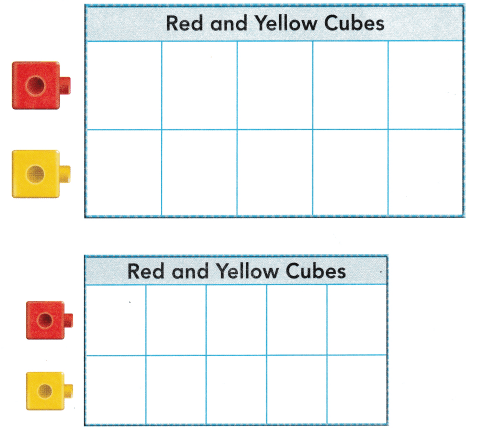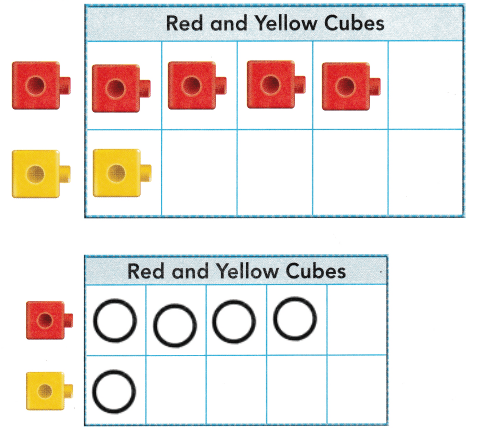Explanation:
John has 4 red cubes and one yellow cube
placed the cubes according to the john cubes.
and drawn the circles.

Directions
Look at the graph at the top of the page. Listen to the problem. Use cubes to show the problem. Look at the graph at the bottom of the page. Draw circles to show the cubes at the top of the page.

Share and Show

Question 1.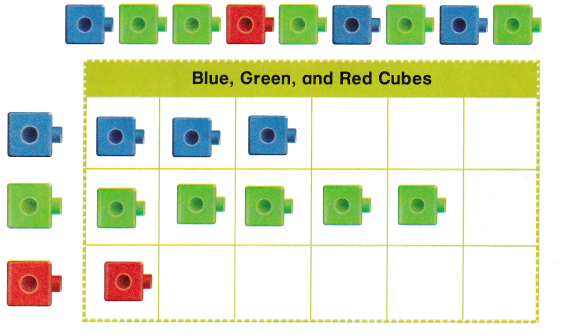Explanation:
There are 5 green cubes, 1 red cube
and 3 blue cubes.

Question 2.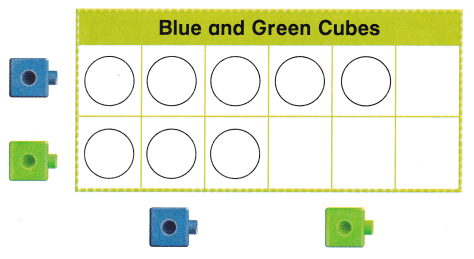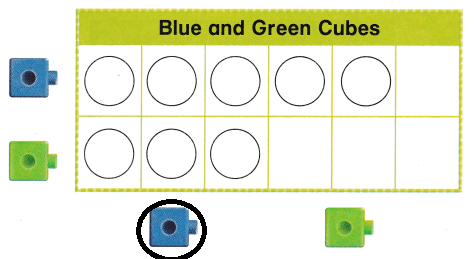Explanation:
There are 5 blue cubes and 3 green cubes
as blue cubes are more
so, circled the blue cube.

Directions
1. Use the cubes shown of the top of the page Sort the cubes and move them to the graph. Draw circles to show the cubes. 2. Read the graph, Which color has fewer cubes? Circle the cube below the graph.

Question 3.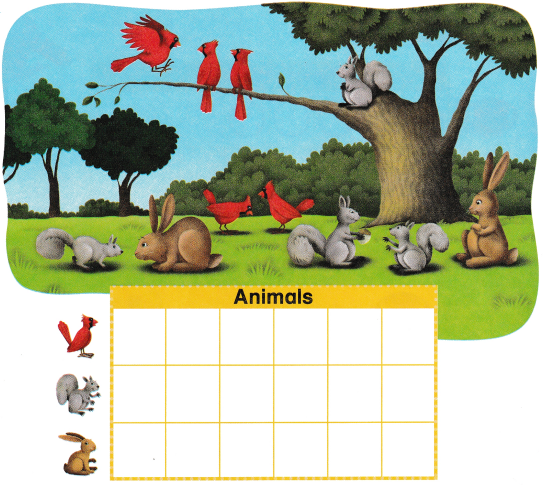Explanation:
There are 5 red birds 3 squirrels and 2 rabbits
so, drawn circles according to the animals.

Question 4.Explanation:
There are 5 red birds 3 squirrels and 2 rabbits
so, written numbers according to the animals.

Directions
3. Look at the picture. How many of each animal are there’ Draw circles in the graph to show the number of each animal. 4. Write how many of each animal.

Home Activity

• Have your child draw another rabbit in the picture at the top of the page. Ask your child to change the graph to match the picture and explain why the graph changed.

Problem Solving

Question 5.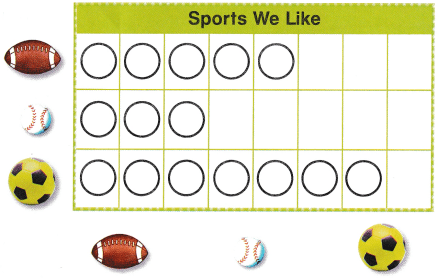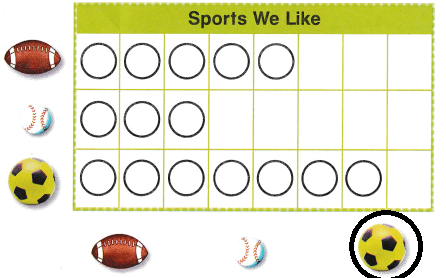Explanation:
There are 7 yellow balls
so, circled the yellow ball as it is more than the white balls and red balls.

Question 6.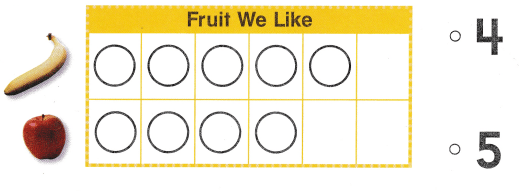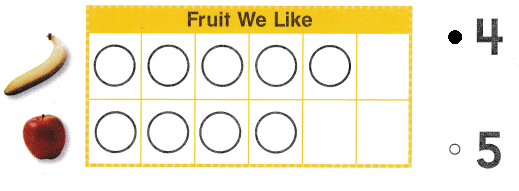Explanation:
4 children likes apples
so, marked the number 4.

Directions
5. Read the picture graph. Circle the ball to show the sport that the most children like. 6. Choose the correct answer. How many children like apples?

### Texas Go Math Kindergarten Lesson 20.4 Homework and Practice

Question 1.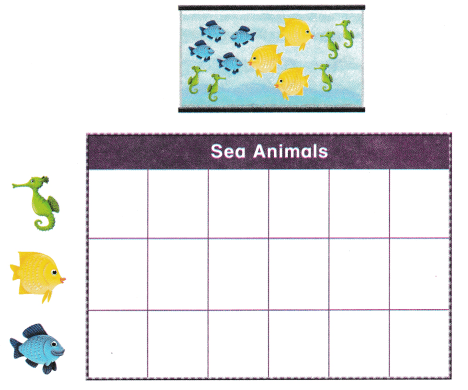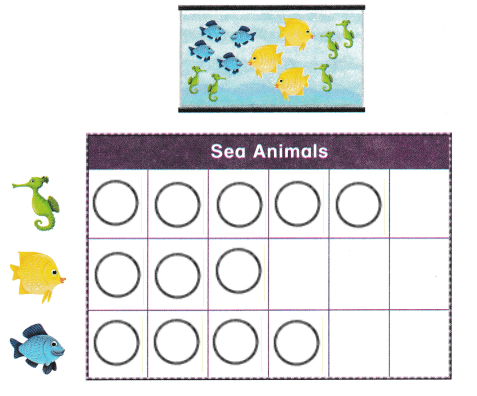Explanation:
There are 5 sea horses 3 yellow fishes
and 4 blue fishes
so, drawn circles according to there numbers.

Question 2.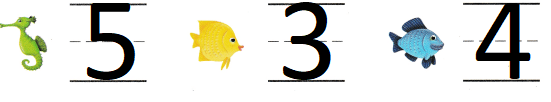Explanation:
There are 5 sea horses 3 yellow fishes
and 4 blue fishes

Directions
1. Look at the fish tank. How many of each sea animal are there? Draw circles in the graph to show the number of each sea animal.

Texas Test Prep

Lesson Check

Question 3.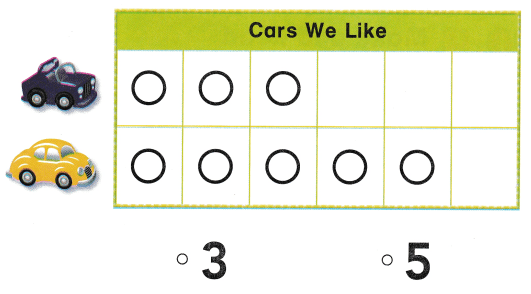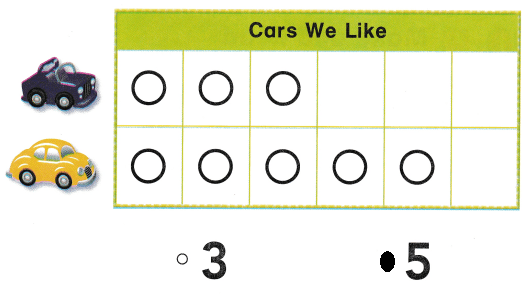Explanation:
There are 5 yellow cars and 3 black cars
so, marked the number 5

Question 4.